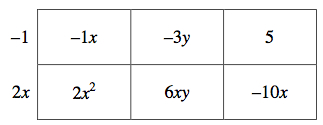Home > INT2 > Chapter 4 > Lesson 4.1.1 > Problem4-7

4-7.

Write the area of the rectangle below as a sum and as a product. Homework Help ✎

 $-1x$ $-3y$ $5$ $2x^2$ $6xy$ $-10x$

Identify the greatest common factor in each row.

Identify the greatest common factor in each column. Watch your signs.

As a product area = (2x − 1)(x + 3y − 5)
As a sum area = 2x² − 11x + 6xy − 3y + 5